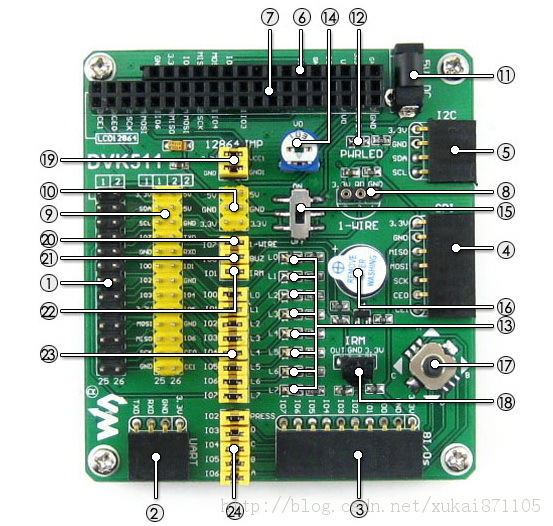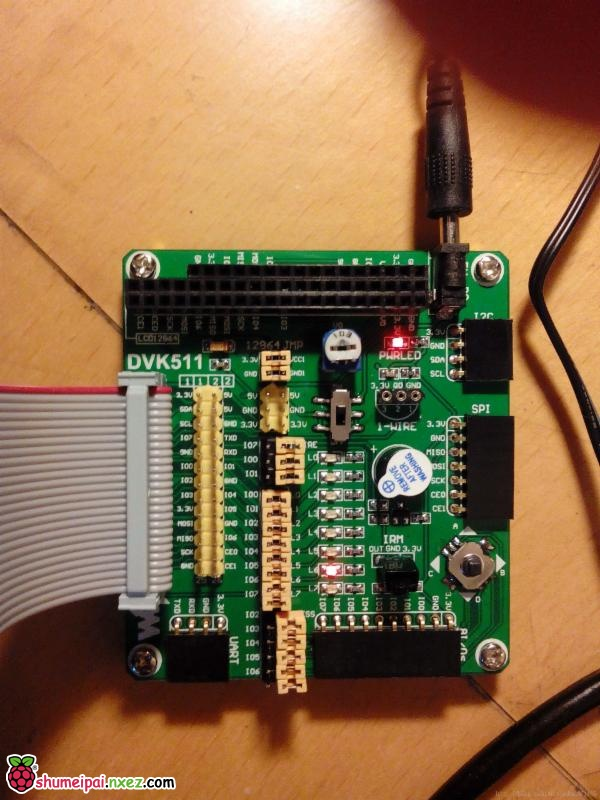python GPIO

wiringPi

BCM2835 C Library

1.硬件准备2.程序实现
2.1 Python

 `#!/usr/bin/env python` `# -*- coding: utf-8 -*-` `import` `RPi.GPIO as GPIO` `import` `time` `GPIO.setmode(GPIO.BOARD)` `# need to set up every channel which are using as an input or an output` `GPIO.setup(``11``, GPIO.OUT)` `while` `True``:` `GPIO.output(``11``, GPIO.HIGH)` `time.sleep(``1``)` `GPIO.output(``11``, GPIO.LOW)` `time.sleep(``1``)`

 `sudo` `python led.py`

2.2 wiringPi

 `#include ` `main ()` `{` `wiringPiSetup () ;` `pinMode (0, OUTPUT) ;` `for` `(;;)` `{` `digitalWrite (0, HIGH) ; delay (500) ;` `digitalWrite (0,  LOW) ; delay (500) ;` `}` `}`

 `gcc` `-Wall -o blink blink.c -lwiringPi`

 `sudo` `.``/blink`

2.3 BCM2835 C Library

 `#include ` `// Blinks on RPi Plug P1 pin 11 (which is GPIO pin 17)` `#define PIN RPI_GPIO_P1_11` `int` `main(``int` `argc, ``char` `**argv)` `{` `if` `(!bcm2835_init())` `return` `1;` `// Set the pin to be an output` `bcm2835_gpio_fsel(PIN, BCM2835_GPIO_FSEL_OUTP);` `// Blink` `while` `(1)` `{` `bcm2835_gpio_write(PIN, HIGH);` `bcm2835_delay(100);` `bcm2835_gpio_write(PIN, LOW);` `bcm2835_delay(100);` `}` `bcm2835_close();` `return` `0;` `}`

 `gcc` `-o blink blink.c -l bcm2835`

 `sudo` `.``/blink`

3.未来技术